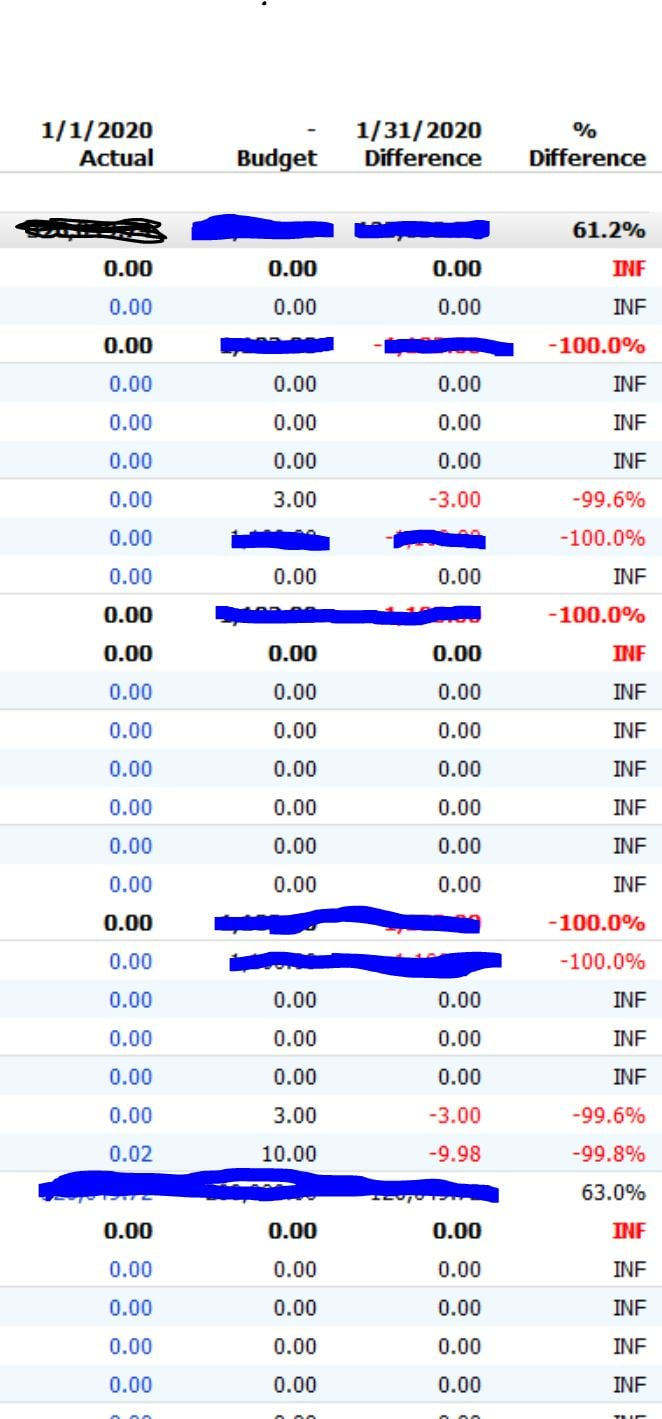# Difference as %

Quicken Premiere 2020, R24.11, Build 27.1.24.11.   A feature that was added was that in comparison reports you could now have the difference between the comparisons shown as a % difference.  (See Below).  I have four questions about the feature.  First, when searching "Help" for this new feature there is very little useful information.  Second, what does INF mean?  I looked in HELP but nothing that I could find.  Third, INF appears when the actual and budget are 0.00, instead of INF shouldn't the percentage be 0.00. Finally, in several instances where the Actual is 0.00, the Budget is an amount say 3.00 and the difference is 3.00 the percentage difference is -100% BUT in another instance (see below) where the actual is 0.00, the budget is 3.00 and the difference is 3.00 the difference is -99.6%  Shouldn't this be -100% as well like the other instances where the actual is 0.00 and the Actual and Difference are the same?Tagged: